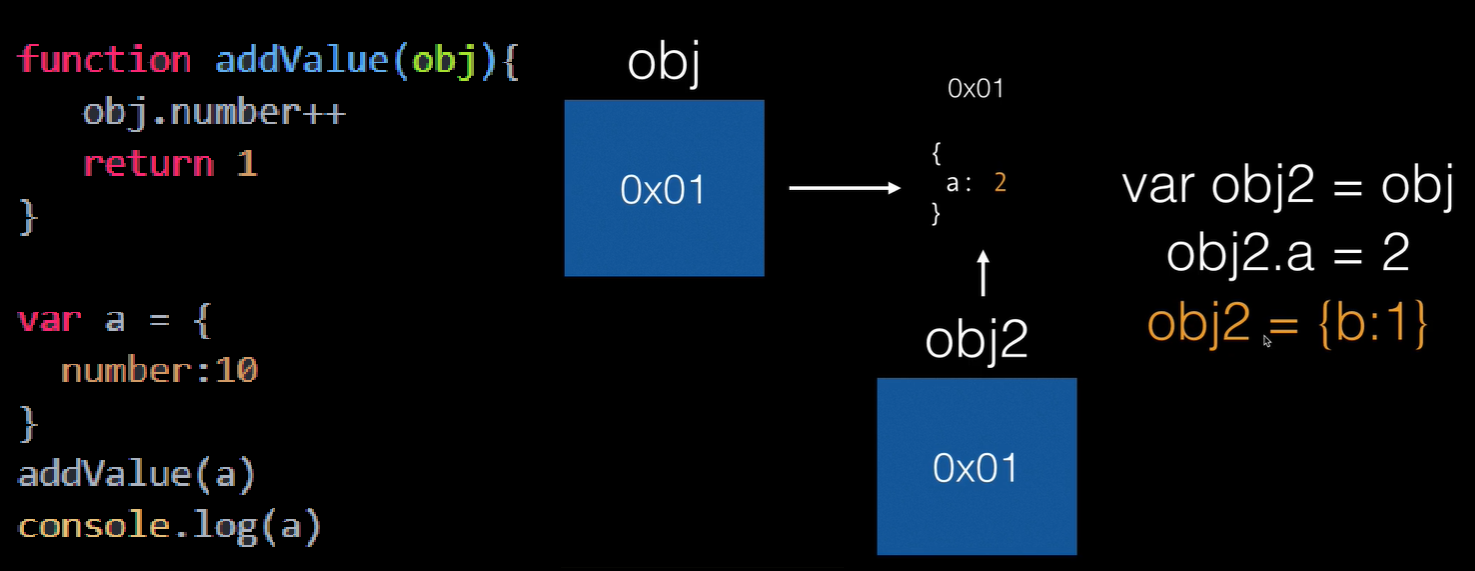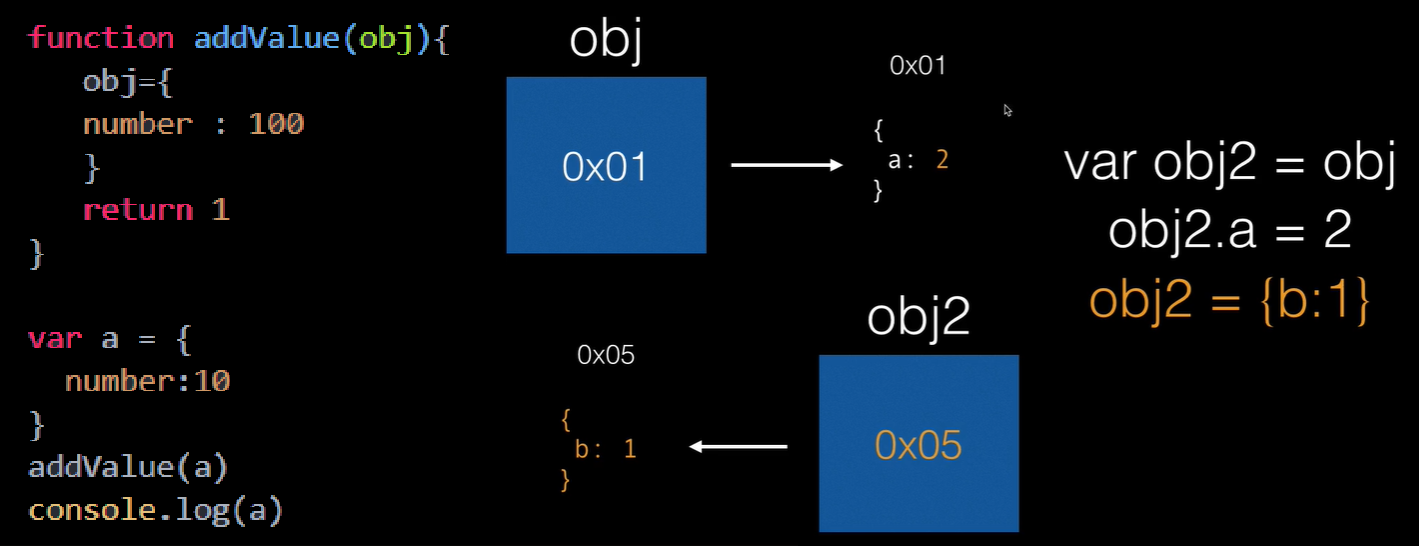# [ JavaScript 06 ] function 傳參數的運作機制

function swap(a,b){
var temp = a
a = b
b = temp
console.log('a,b=',a,b)
}
var number1 = 10
var number2 = 20
console.log('1',number1,number2)

swap(number1,number2)

console.log('2',number1,number2)

• 解說function swap裡面發生的事，為何console.log('2',number1,number2)的值不會改變 :
var a = number1
var b = number2
a 和 b 是複製來的
它的記憶體跟 number1,number2 的位置是不一樣
傳進去後，a 和 b 的確變了，但不會影響到外面 number1,number2 的值
值（value）一樣，但是完全兩個不一樣的變數

value 改變的例子

function addValue(obj){
obj.number++
return 1
}

var a = {
number:10
}

console.log(a)

{number: 11}


a 印出來是 11 而不是 10

• 不會改變 (主要的原始資料類型)
數字(Number)
字串(String)
布林(Boolean)

• 會改變 (複合型(composite)或參考型(reference)的資料類型)
陣列(Array)
物件(Object)

value 又不會改變的例子 我跳出去，我又跳進來 (~￣▽￣)~~ .... ~(￣▽￣~)

function addValue(obj){
obj={
number : 100
}
return 1
}

var a = {
number:10
}

console.log(a)

{number: 10}


value 改變的例子說明:value 不會改變的例子說明:a 是物件(Object)，要小心寫的時候，裡面多打什麼就會什麼印出來

function addValue(obj){
obj.test=1
return 1
}

var a = {
number:10
}

console.log(a)

{number: 10, test: 1}


• pass by value
• pass by reference
• pass by sharing

pass by sharing 屬於在 pass by value 底下

Java 中 Call by value，指的是傳遞參數時，一律傳遞變數所儲存的值，無論是基本型態或是類別宣告的型態都一樣，Java 中不允許處理記憶體位址，所以用了「參考」這個名稱來作為解釋類別型態所宣告的變數之行為，但這邊的「參考」與 C++ 中所稱之「參考」，是完全不相同的行為，更不會有 C++ 中參數的傳值、傳參考、return 的傳值、傳參考的 Call by reference 行為。